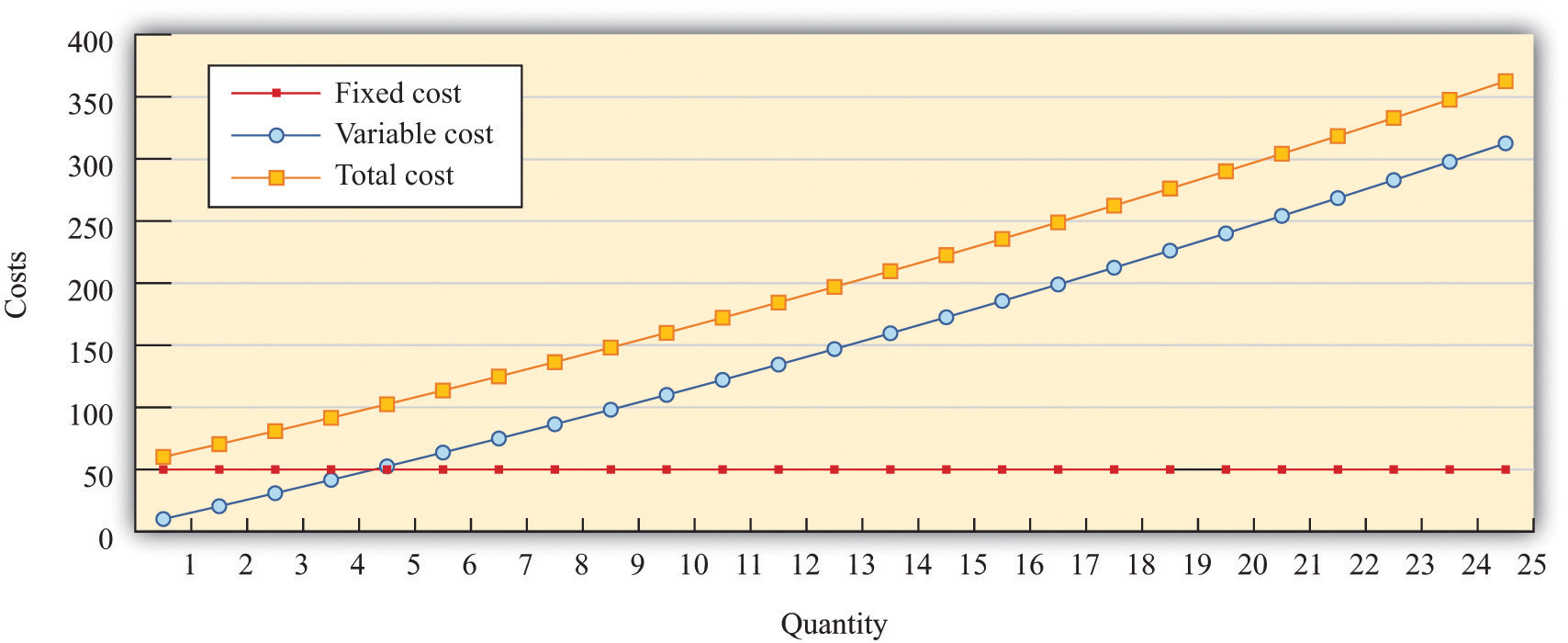This is “Costs of Production”, section 31.14 from the book Theory and Applications of Economics (v. 1.0). For details on it (including licensing), click here.

For more information on the source of this book, or why it is available for free, please see the project's home page. You can browse or download additional books there. To download a .zip file containing this book to use offline, simply click here.

Has this book helped you? Consider passing it on:
Creative Commons supports free culture from music to education. Their licenses helped make this book available to you.
DonorsChoose.org helps people like you help teachers fund their classroom projects, from art supplies to books to calculators.

## 31.14 Costs of Production

The costs of production for a firm are split into two categories. One type of cost, fixed costs, is independent of a firm’s output level. A second type of cost, variable costs, depends on a firm’s level of output. Total costs are the sum of the fixed costs and the variable costs.

The change in costs as output changes by a small amount is called marginal cost. It is calculated as follows:

Because fixed costs do not depend on the quantity, if we produce one more unit, then the change in total cost and the change in the variable cost are the same. Marginal cost is positive because variable costs increase with output. Marginal cost is usually increasing in the level of output, reflecting the diminishing marginal product of factors of production.

For example, suppose that total costs are given by

total costs = 50 + 10 × quantity.

Here the fixed cost is 50, and the variable cost is 10 times the level of output. In this example, marginal cost equals 10. These costs are shown in Table 31.5.

Table 31.5

Output Fixed Cost Variable Cost Total Cost
0 50 0 50
10 50 100 150
20 50 200 250
50 50 500 550

We sometimes divide fixed costs into two components: entry costs, which are the one-time fixed costs required to open a new business or set up a new plant, and fixed operating costs, which are the fixed costs incurred regularly during the normal operation of a business.

Some costs are sunk costs; once incurred, these costs cannot be recovered. Such costs should be ignored in forward-looking business decisions. Other costs are partially or fully recoverable costs. For example, if a firm purchases an asset that can be resold, then the cost of that asset is recoverable.

Figure 31.11 Cost MeasuresFigure 31.11 "Cost Measures" shows these various measures of costs. It is drawn assuming a fixed cost of 50 and variable costs given by

variable costs = 10 × quantity + 0.1 × quantity2.

For this example, marginal cost is positive and increasing.

## Key Insights

• Fixed costs are independent of the level of output, whereas variable costs depend on the output level of a firm.
• Pricing decisions depend on marginal costs.
• Decisions to enter and/or exit an industry depend on both fixed and variable costs.